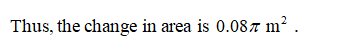# The radius of a circle is increased from 2.00 to 2.02 m. a. Estimate the resulting change in area. b. Express the estimate as a percentage of the circle’s original area.

Question
64 views

The radius of a circle is increased from 2.00 to 2.02 m. a. Estimate the resulting change in area. b. Express the estimate as a percentage of the circle’s original area.

check_circle

Step 1

a) It is given that; radius of the circle is increased from 2 to 2.02m.Obtain the change in area,

Step 2Step 3

b) Estimate the percentage of th...

### Want to see the full answer?

See Solution

#### Want to see this answer and more?

Solutions are written by subject experts who are available 24/7. Questions are typically answered within 1 hour.*

See Solution
*Response times may vary by subject and question.
Tagged in
MathCalculus

### Other